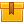# Electrical Schematic Symbols – Logic and Output Devices

## Prerequisites

Introduction to Electrical Schematics is recommended.

## Description

This is the third lesson in the iKNOW Electrical Print Reading Library. This lesson defines the function of logic and output elements of a control circuit and presents the symbols for various logic and output devices.

## Objectives

Describe the function of the logic element of a control circuit. Describe the function of the output element of a control circuit. Identify the symbol for a relay and the associated contacts. Identify various logic symbols and state how they are used. Identify the symbol for a motor starter and state how it is used. Identify various output symbols and state how they are used.

## ContentThis is the third lesson in the iKNOW™ Electrical Print Reading Library.
This lesson defines the function of logic and output elements of a
control circuit and presents the symbols for various logic and output
devices.

•Electrical Schematic Symbols – Logic and Output Devices

0
0 Reviews